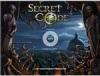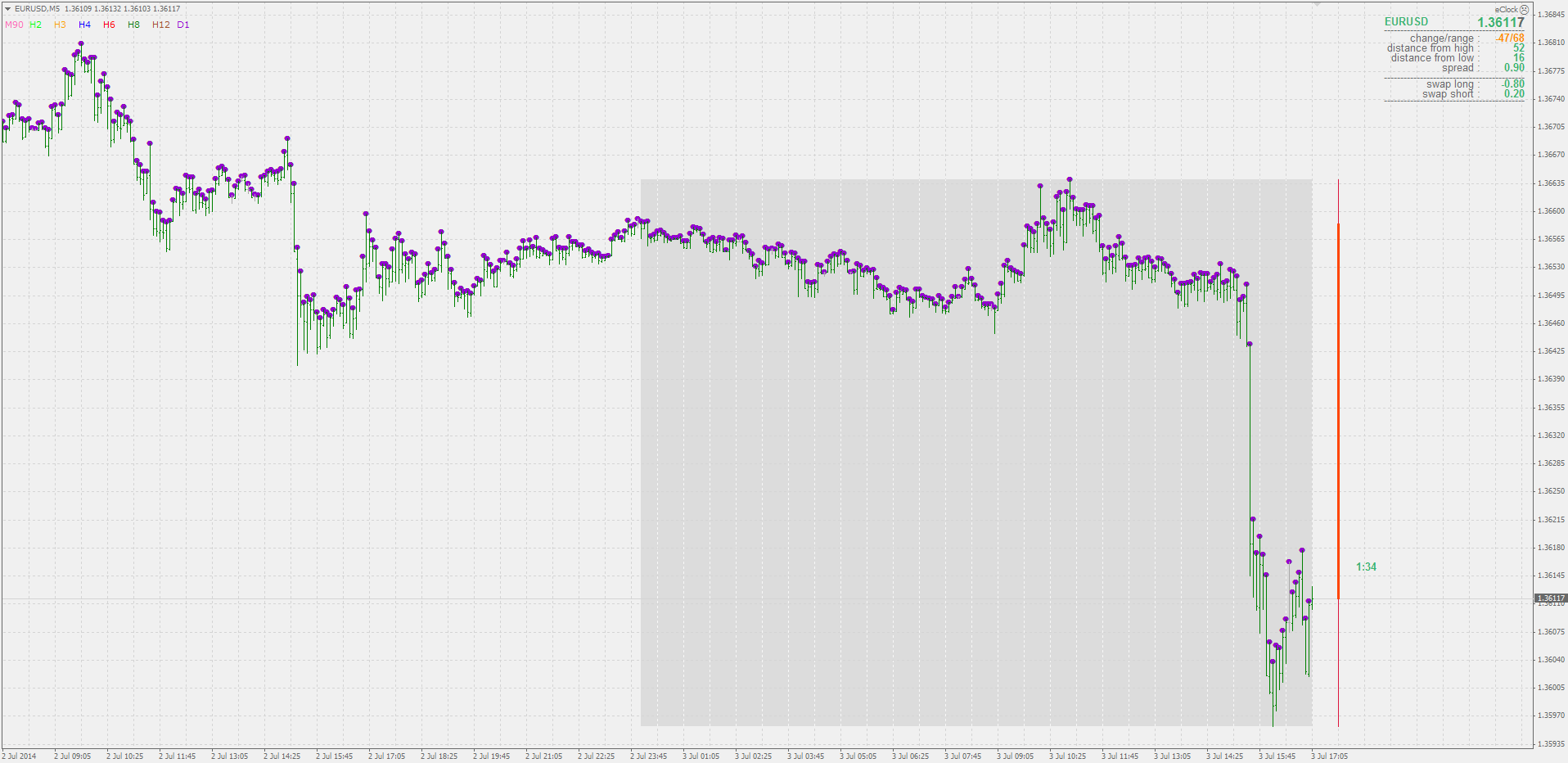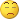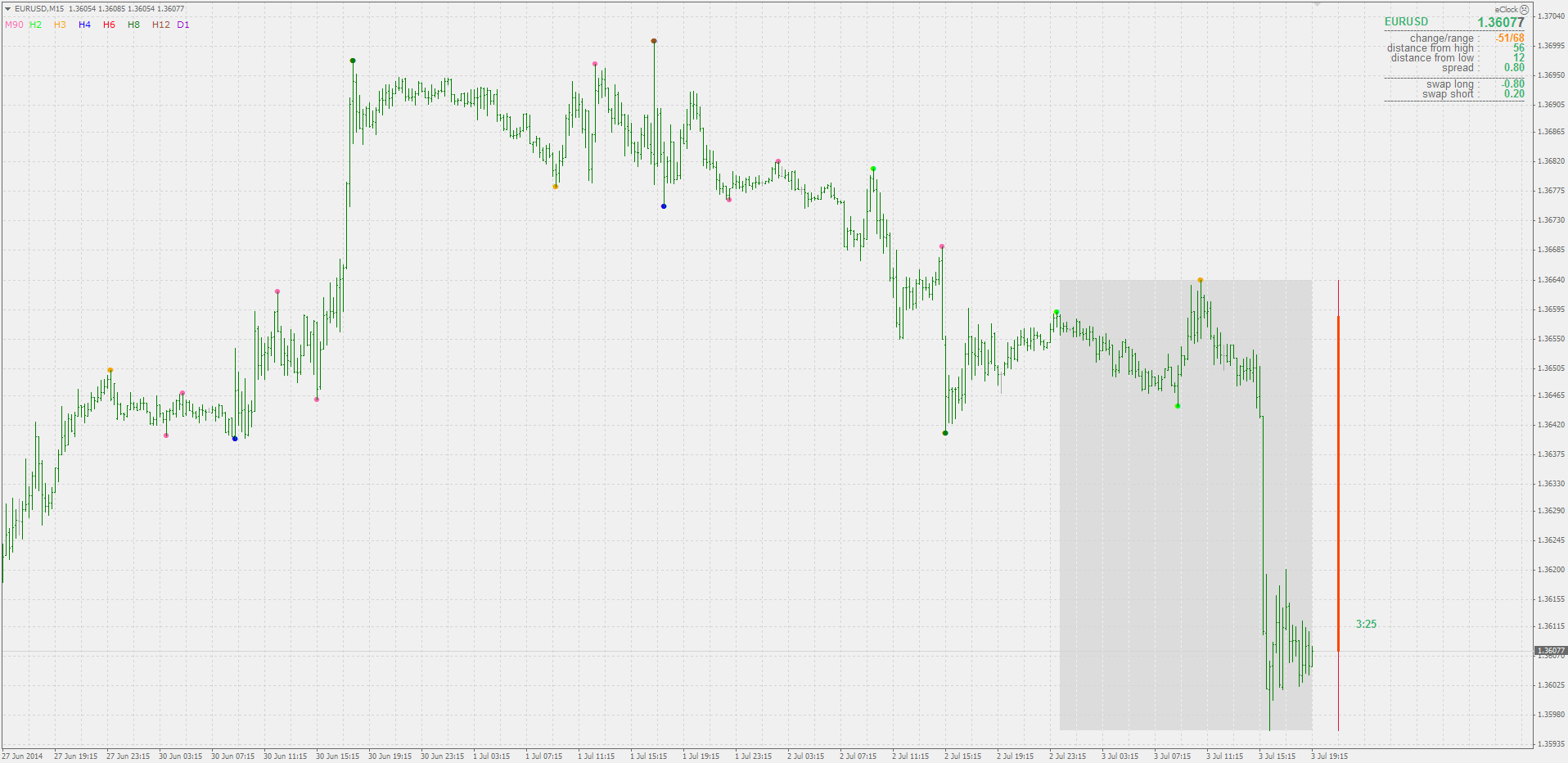# Indicators with alerts/signal - page 822153865

emmany4:

emmany4

That indicator is looking one future bar ahead. That is why it does not alert on a current bar (it does not "know" the value of the future bar for the current bar) but only on a first closed bar587

mrtools:
Hello Camisa, it's a decompiled indicator, any chance you have the original.

hello MrTools, I attached the .mq4 and not the ex3 version so you should be able to open it in metaeditor?

anyway, here is the code:

#property indicator_chart_window

#property indicator_buffers 2

#property indicator_color1 DodgerBlue

#property indicator_color2 Red

extern int uMA_Length = 11;

extern int uREI_Length = 3;

extern double uREI_OB = 85.0;

extern double uREI_OS = -65.0;

datetime G_time_100;

double G_ibuf_104[];

double G_ibuf_108[];

// E37F0136AA3FFAF149B351F6A4C948E9

void init() {

IndicatorDigits(Digits);

SetIndexBuffer(0, G_ibuf_108);

SetIndexStyle(0, DRAW_ARROW);

SetIndexArrow(0, 159);

SetIndexEmptyValue(0, 0.0);

SetIndexBuffer(1, G_ibuf_104);

SetIndexStyle(1, DRAW_ARROW);

SetIndexArrow(1, 159);

SetIndexEmptyValue(1, 0.0);

IndicatorShortName("Trend Turn (" + uMA_Length + "," + uREI_Length + "," + DoubleToStr(uREI_OB, 0) + "," + DoubleToStr(uREI_OS, 0) + ")");

G_time_100 = 0;

}

// EA2B2676C28C0DB26D39331A336C6B92

void start() {

bool Li_0;

double ima_4;

double Lda_12;

if (Time != G_time_100) {

Li_0 = TRUE;

if (G_time_100 == 0) {

Li_0 = Bars - MathMax(uMA_Length, uREI_Length) - 1.0;

ArrayInitialize(G_ibuf_104, 0.0);

ArrayInitialize(G_ibuf_108, 0.0);

}

G_time_100 = Time;

for (int Li_16 = Li_0; Li_16 >= 1; Li_16--) {

ima_4 = iMA(NULL, 0, uMA_Length, 0, MODE_SMA, PRICE_CLOSE, Li_16);

Lda_12 = f0_0(Li_16);

Lda_12 = f0_0(Li_16 + 1);

if (Close[Li_16] uREI_OS && Lda_12 < uREI_OS) G_ibuf_108[Li_16] = Low[Li_16];

if (Close[Li_16] > ima_4 && Lda_12 uREI_OB) G_ibuf_104[Li_16] = High[Li_16];

}

}

}

// 1FA5B2436F15D3CDBF65E8F1FBA6CC74

double f0_0(int Ai_0) {

double Ld_4 = 0.0;

double Ld_12 = 0.0;

double Ld_20 = 0.0;

for (int count_28 = 0; count_28 < uREI_Length; count_28++) {

Ld_12 += High[Ai_0 + count_28] - (High[Ai_0 + count_28 + 2]) + (Low[Ai_0 + count_28] - (Low[Ai_0 + count_28 + 2]));

Ld_20 += MathAbs(High[Ai_0 + count_28] - (High[Ai_0 + count_28 + 2])) + MathAbs(Low[Ai_0 + count_28] - (Low[Ai_0 + count_28 + 2]));

}

if (Ld_20 > 0.0) Ld_4 = Ld_12 / Ld_20;

return (100.0 * Ld_4);

}898

Something wrong with it (probably wrong with build 660 I use for testing)

See what is it showing to me :Attached indicator (EX4) is working!! Please check the image how it looks on my terminal (build 646)!

Files:153865

secretcode:
Attached indicator (EX4) is working!! Please check the image how it looks on my terminal!

I know

But I can not change the ex4. When I compile the source I get that nonsense. Checking it using the strict but so far no result132

Что-то не так с ним (вероятно, неправильно со встроенным 660 я использую для тестирования)

Посмотрите, что он показывает мне: [/ QUOTE]Try this timeframe to correct and alert to do.

Files:
fd_1.png 16 kb153865

Младен; 689864:
Что-то не так с ним (вероятно, неправильно со встроенным 660 я использую для тестирования)

Посмотрите, что он показывает мне: [/ QUOTE]Try this timeframe to correct and alert to do.

The last one is OK

PS: 16 possible types of alerts. Is that what you had in mind?Files:
ok.gif 70 kb132

Mladen,do alert on every timeframe so that you can individually enable or disable alert. For example, only the h1 and d1 is enabled.

Files:
fd....png 18 kb164

samuelkanu Why don't you use MACD zero cross alerts (they are virtually the same)?153865

samuelkanu:

samuelkanu

Any macd will do. Difference between main values are going to be marginal27

Младен; 689864:
Что-то не так с ним (вероятно, неправильно со встроенным 660 я использую для тестирования)

Посмотрите, что он показывает мне: [/ QUOTE]Try this timeframe to correct and alert to do.

sorry,i don't know how to understand this,can you help me?